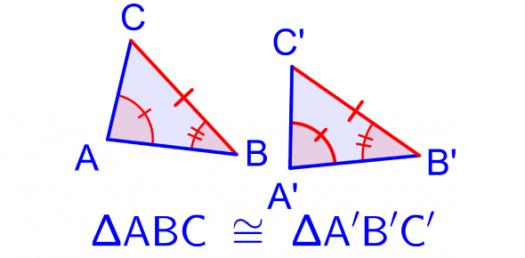# Geometry Trivia Questions Test! Basic Quiz

5 Questions | Attempts: 179SettingsAre you looking for some geometry trivia questions to help increase your ability to solve some of the common problems you will come across in the study? If you said yes, you are in luck as the quiz below is exactly what you need to refresh your memory. Give it a go and keep a lookout for other quizzes like it as practice makes perfect!

• 1.
Find the axis intercepts and gradient of the like with the equation 5x-2y=10.
• A.

X-intercept = 4, y-intercept = -4 and gradient - 6/9

• B.

X-intercept = 4, y-intercept = -32 and gradient - 64/1

• C.

X-intercept = 4, y-intercept = -8 and gradient - 6/9

• D.

X-intercept=2, y intercept= -5 and gradient = 5/2

• E.

X-intercept = 1, y-intercept = -8 and gradient - 62/3

• 2.
Determine the equations of the following lines:a) gradient -3, y-intercept 4        b)through the points(-3,4) and (3,1).
• A.

Y=3x+9

• B.

Y= -3x+4

• C.

Y= x+2

• D.

Y= -7-6x

• E.

Y=5x+4

• 3.
Find the distance between P(-4,7) and Q(-1,3).
• A.

5 units

• B.

8units

• C.

15units

• D.

12 units

• E.

10units

• 4.
The radius of a circle is 13cm and AB is a chord that is at a distance of 12cm from the center. The length of the ladder is:
• A.

35 cm

• B.

17.5 cm

• C.

25 cm

• D.

10 cm

• 5.
Katie keeps her earrings in a beautiful glass case which has six sides. What is the name of a polygon with six sides?
• A.

Octagon

• B.

Hexagon

• C.

Nonagon

• D.Back to top